#### You may also like### Circles Ad Infinitum

A circle is inscribed in an equilateral triangle. Smaller circles touch it and the sides of the triangle, the process continuing indefinitely. What is the sum of the areas of all the circles?### Building Tetrahedra

Can you make a tetrahedron whose faces all have the same perimeter?### Ladder and Cube

A 1 metre cube has one face on the ground and one face against a wall. A 4 metre ladder leans against the wall and just touches the cube. How high is the top of the ladder above the ground?

# Always Perfect

##### Age 14 to 18Challenge Level

Part 1(a): Take two numbers that differ by 2, multiply them together and add 1 (so for example $5 \times 7 + 1 = 36$).

Nikita from Jersey College for Girls noticed a pattern: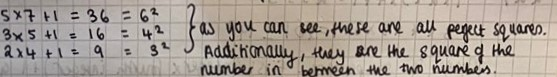Charles from Rugby School in the UK, Rin from Wren Academy in England, Mahdi from Mahatma Gandhi International School in India, Airi from Japan, Isabel from England, Nikita and David all proved Nikita's rule using algebra (Claire's method). This is Airi's work:

Let the two numbers be $x$ and $x + 2.$
Multiplying them and then adding $1$ results in $x(x + 2) + 1 = x^2 + 2x + 1.$ This is equal to $(x+1)^2.$

So the result is always equal to the square of the arithmetic mean of the two numbers. (The mean of the two numbers is $\frac {x+(x+2)}2=x+1$).

Mahdi's algebra was different:

Interestingly, if we let the average (or middle number) be $n$, then the two numbers which differ by $2$ will be $n-1,n+1.$

Multiplying the two and adding $1$ gives this:

$(n-1)(n+1) + 1 = n^2 - 1 + 1 = n^2$

A nice application of difference of two squares.

Part 1(b): Take two numbers that differ by 4, multiply them together and add 4 (so for example $5 \times 9 + 4 = 49$).

Nikita used some examples to find a pattern: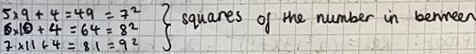Mahdi used a diagram like Charlie's diagram, where the two numbers were $n$ and $n+4$ so the "number in between" would be $n+2$: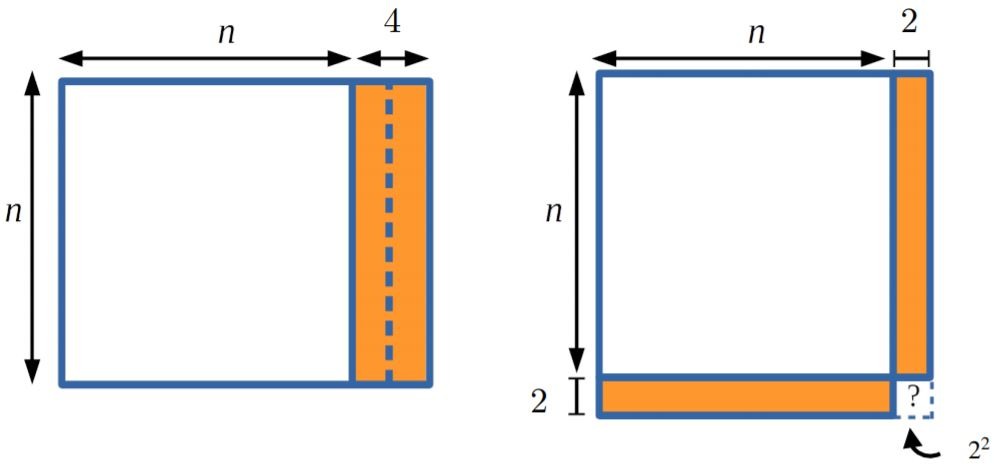Siddharth from Bishop Vesey's Grammar School, Sutton Coldfield in the UK, Charles, David, Nikita, Rin, Isabel, Airi and Mahdi also used algebra to prove the rule. This is Siddharth's work:

We can express the two numbers which have a difference of $4$ as $x$ and $x+4.$ We need to multiply the numbers and then add $4$ on. Using our definition of the two numbers, we can write this process algebraically as $x^2 + 4x + 4.$

What I notice is that this process gives the square of the mean of the two numbers, $\left( \frac {2x+4}2\right)^2.$ This can be simplified to $x^2 + 4x + 4.$ This proves that if you multiply any two numbers which have a difference of four and if you add $4$ on, the result is always the square of the mean of the two numbers.

Again, Mahdi used different algebra:

Armed with the difference of squares ovservation, I took the two numbers to be $n-2, n+2$

Multiplying the two and adding $4$ (because $2^2$) gives this:

$(n-2)(n+2) + 4 = n^2 - 4 + 4 = n^2$

Here $n$ is the average of the two numbers that I took. For example, if the numbers which differ by four are $5$ and $9,$ then the addition of their product and $4$ would be $7$ squared.

Part 1(c): Take two numbers that differ by 6, multiply them together and add 9

Hannah from Dulwich College Beijing in China made this observation: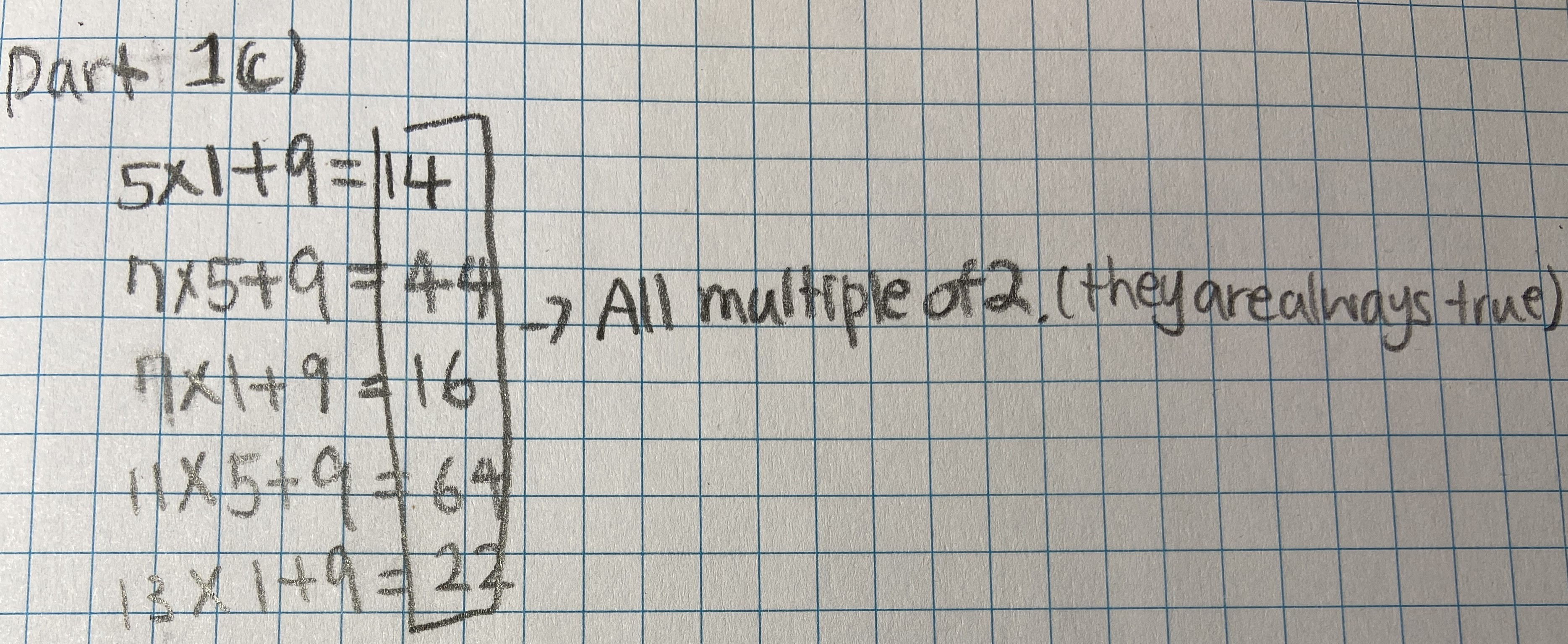However, what if the two numbers are 2 and 8?

Nikita found a different pattern: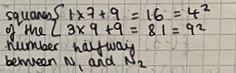Charles, David, Nikita, Ria, Isabel and Airi proved the pattern continues. This is Charles's work: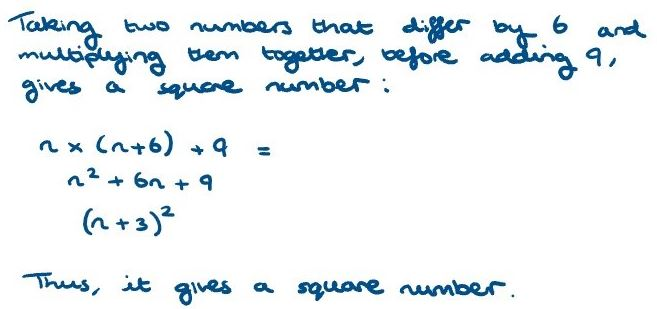Airi concluded:

So the result is always equal to the square of the arithmetic mean of the two numbers.

Part 1(d): Looking at your results for parts (a), (b) and (c) can you predict what you will have to add to the product of two numbers that differ by $8, 10, 12, ..., 2k$ in order to get a square number?

Rin and Nikita tried some examples and proved their results. This is Rin's work:

Difference of $8$:
$n(n+8)+a=(n+4)^2$
$a=4^2=16$

Difference of $10$:
$n(n+10)+b=(n+5)^2$
$b=5^2=25$

Difference of $12$:
$n(n+12)+c=(n+6)^2$
$c=6^2=36$

Difference of $2k$:
$n(n+2k)+m=(n+k)^2$
$m=k^2$

Multiplying two numbers with a difference of $2k$ and adding $k$ to the answer will give the square of these two numbers' mean.

$n(n+2k)+k^2$
$=n^2+2kn+k^2$
$=(n+k)^2$

Sanika from PSBMMS,OMR in India, David, Nikita, Isabel and Airi all wrote similar proofs.

Charles expressed the numbers in a slightly different way: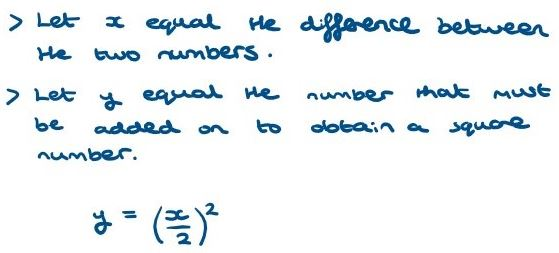Once again, Mahdi used different algebra and a diagram:

(1) My method:

Since the two numbers differ by $2k$, the numbers can be $n-k, n+k$

Multiply the two numbers and add $k^2$ $$\begin{split}(n-k)(n+k) + k^2 &= n^2 - k^2 + k^2\\&=n^2\end{split}$$Note: the $n$ in my method is different to the $n$ in Claire's approach and Charlie's method

(2) by Charlie's method: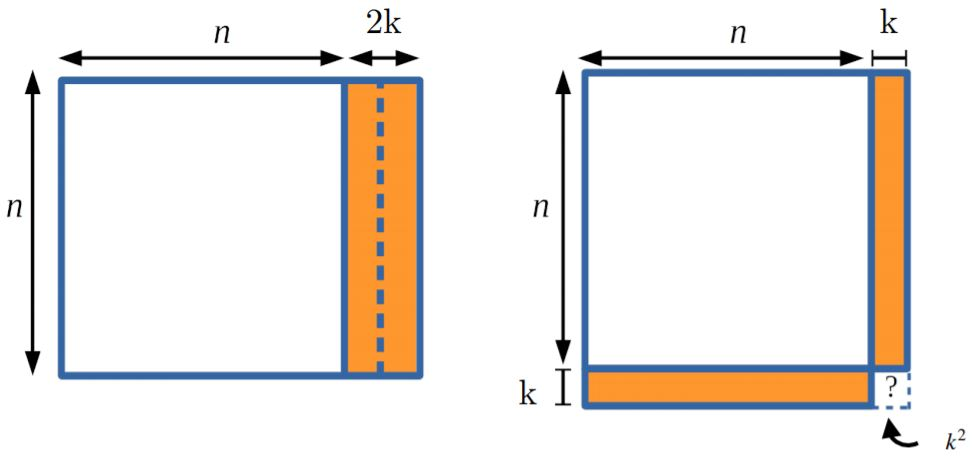Part 2(a): Consider what happens when $1$ is added to the product of four consecutive numbers, for example $2\times 3 \times 4 \times 5 + 1 = 121$.

As Charlie suggested, Nikita used some examples to find a rule. Nikita then proved the rule: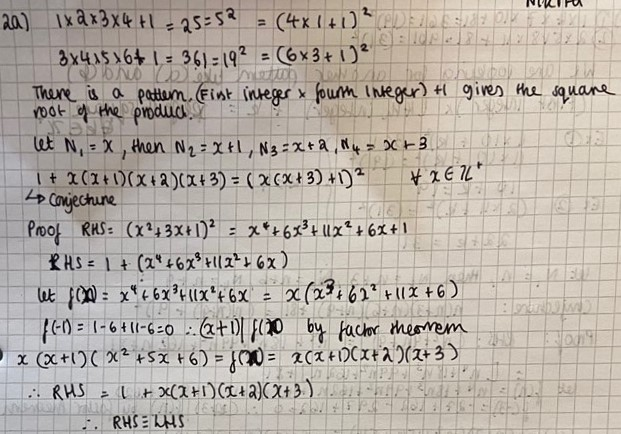Isabel noticed and proved a slightly different rule: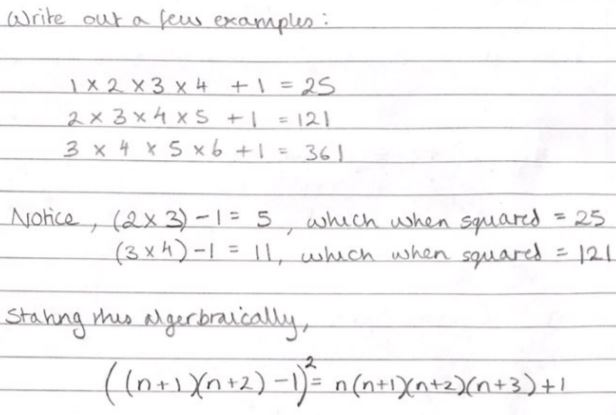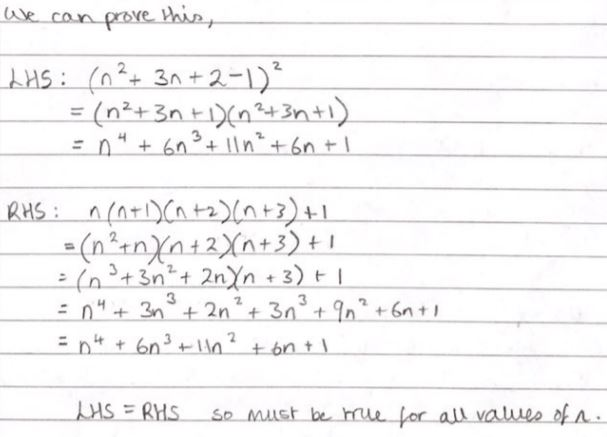David proved the same rule in a different way:Using Claire's approach, Mahdi, Rin and Airi started by using algebra. Rin wrote:

$n(n+1)(n+2)(n+3)+1\\= n(n+3)\times(n+1)(n+2)+1\\=(n^2+3n)(n^2+3n+2)+1\\=(n^2+3n)^2+2(n^2+2)+1\\=\left((n^2+3n)+1\right)^2\\ =(n^2+3n+1)^2$

Airi factoried the final term to get the same result as Nikita (using $x$ instead of $n$):

$=\left(x(x+3)+1\right)^2$

Part 2(b): Take four consecutive even numbers (or four consecutive odd numbers), multiply them together and add 16 (so for example $2\times 4 \times 6 \times 8 + 16 = 400$).

Nikita found and proved a rule: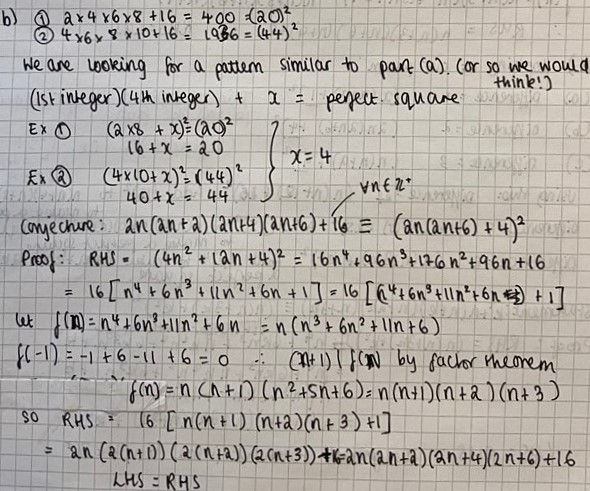Because Nikita's first number is $2n$, Nikita's proof only works when the numbers are even. Airi wrote a similar proof which works when the first number is odd or even:

$\begin{split}x(x+2)(x+4)(x+6) + 16 &= x^4 + 12x^3 + 44x^2 + 48x + 16 \\&= (x^2 + 6x + 4)^2 \\&= \left(x(x+6) + \sqrt{16}\right)^2\end{split}$

Isabel noticed and proved a different rule. Here is Isabel's work: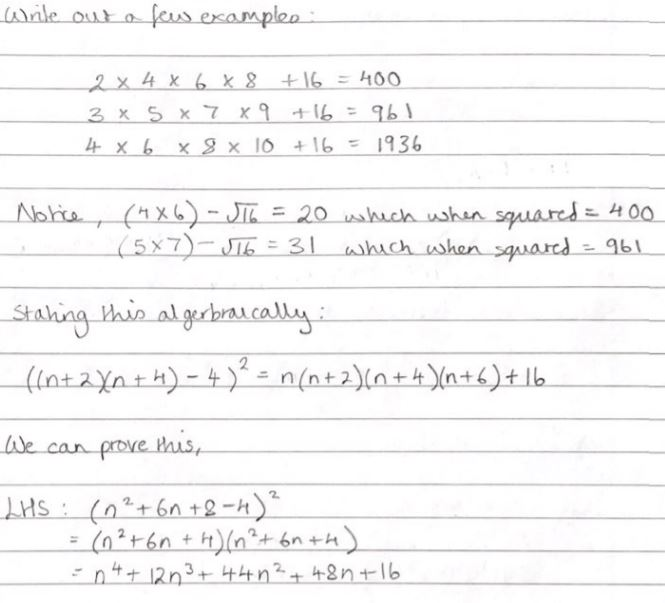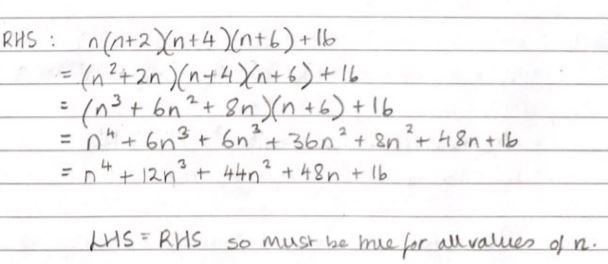David and Sanika found and proved yet another rule. Sanika wrote:

The product of 4 consecutive even/odd numbers is equivalent to $$\begin{split}(x-3)(x-1)(x+1)(x+3)&=(x^2-9)(x^2-1)\\&= x^4-10x^2+9\end{split}$$Since $x^4-10x^2+9+16=x^4-10x^2+25=\left(x^2-5\right)^2$, the product of 4 consecutive odd/even numbers $+16$ will always be a perfect square.

David concluded:

The result will always be the mean of the four numbers, squared, minus 5, then squared again.

Part 2(c): Take four numbers which differ by 3, multiply them together and add 81 (so for example $2\times 5 \times 8 \times 11 + 81 = 961$).

Nikita found and proved a rule similar to her previous rules: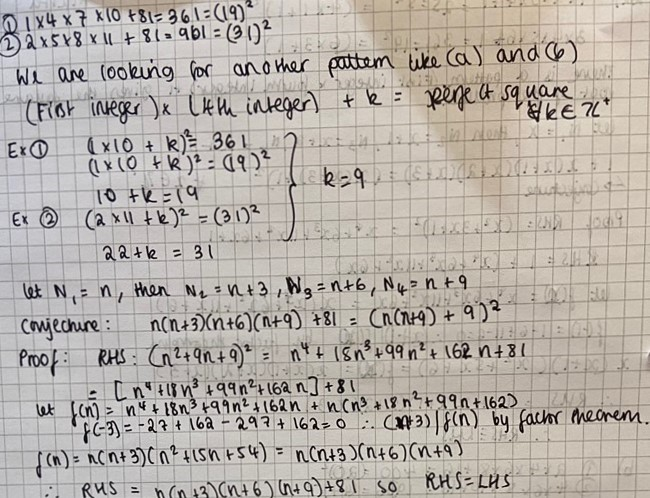Isabel found and proved a rule similar to her previous rules: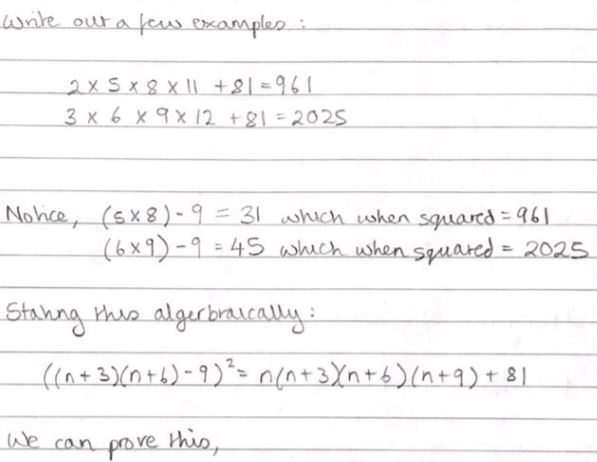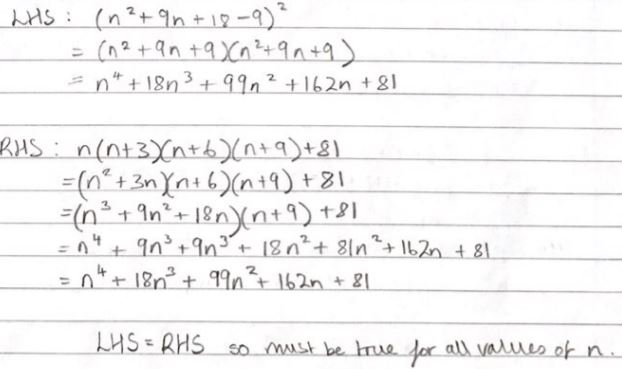David found the same rule as Isabel, but proved it using slightly different algebra:

We have
$(n-3)n(n+3)(n+6)+81\\=\left(n^2-9\right)\left(n^2+6n\right)+81\\=n^4+6n^3-9n^2-54n+81\\ =\left(n(n+3)-9\right)^2$

Sanika found a rule that was inspired by Sanika and David's rule in part (b). This is Sanika's work:

Product of four numbers with a consecutive difference of $3$ is
$(x-4.5)(x-1.5)(x+1.5)(x+4.5)$
[I chose this particular way so that I could get a complete the square sort of expression by simplifying this using the identity $a^2-b^2=(a+b)(a-b)$]
$=(x^2-20.25)(x^2-2.25)$
$=x^4-22.5x^2+45.5625$

Adding $81$ we get $x^4-22.5x^2+126.5625 =(x^2- 11.25)^2$

If the four numbers are whole numbers then $x$ will have a decimal of $0.5,$ in which case $x^2$ will have a decimal value of $0.25.$ Therefore the decimal value will cancel out with the $0.25$ in $11.25.$ Thus, $81$ plus the product of four numbers with a difference of three, will give us a perfect square (provided that the numbers are integers).

Part 2(d): Looking at your results for parts (a), (b) and (c) can you predict what you will have to add to the product of four numbers that differ by $4, 5, 6, ..., k$ in order to get a square number?

Nikita found a general version of the rules she'd proved above: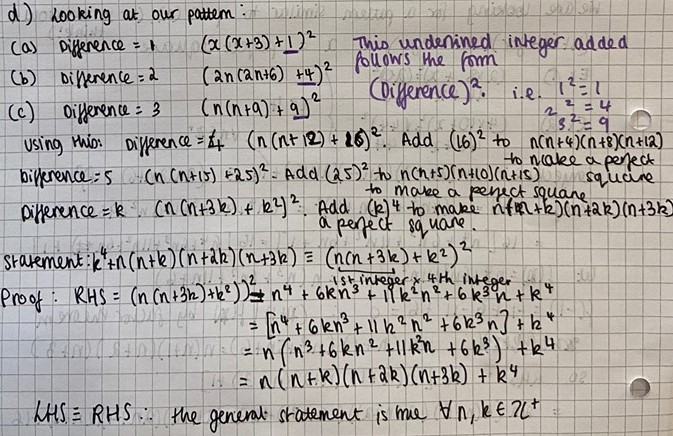Isabel found a general version of the rules that she'd proved above: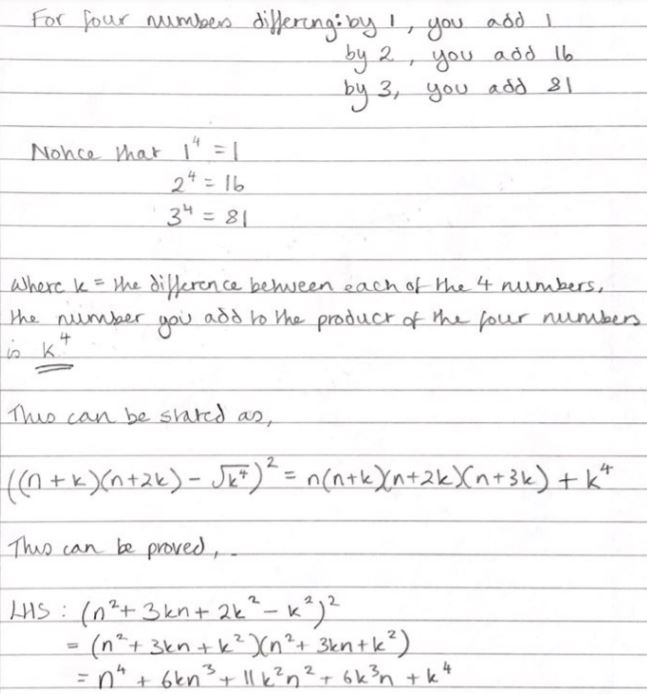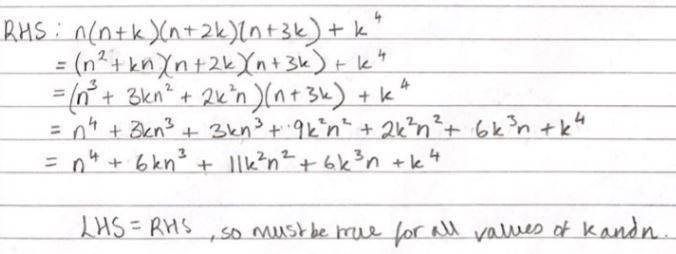David found the same rule as Isabel, but proved it slighty differently:

$(n-k)n(n+k)(n+2k)+k^4\\=\left(n^2-k^2\right)\left(n^2+2n\right)+k^4\\=n^4+2kn^3-k^2n^2-2k^3n+k^4\\=\left(n(n+k)-k^2\right)^2$

Sanika found a general version of the rules that she'd proved above:

If the difference between the numbers is $n$, then the number that must be added to the product of the four numbers is $n^4.$

Proof:
If you are working with a difference of $n$, then you name your sequence as follows:$$\begin{split}\left(x-\frac{3n}2\right)\left(x-\frac{n}2\right)\left(x+\frac{n}2\right)\left(x+\frac{3n}2\right) &= \left(x^2-\frac{9n^2}4\right)\left(x^2-\frac{n^2}4\right)\\&=x^4-\frac{10n^2}4x^2+\frac{9n^4}{16}\end{split}$$For the expression to be a perfect square it has to be in the form$$(a-b)^2 = a^2-2ab+b^2$$(Here $x^4 = a^2$, $2ab=\frac{10n^2}{4}x^2$ and so on)
So this can be expressed as $(x^2-b)^2$ where $b=\frac{-2ab}{-2a}$ which in this case is $\dfrac{\tfrac{-10n^2}{4}x^2}{-2x^2}=\frac{10n^2}8$

$\therefore b^2=\dfrac{100n^4}{64}$

$\Rightarrow$We will have to add $\left(\dfrac{100n^4}{64}-\dfrac{9n^4}{16}\right)=n^4$ to the expression to make it a perfect square.

Mahdi, Rin and Airi also found general expressions. This is Mahdi's work, which is very similar to Rin's work: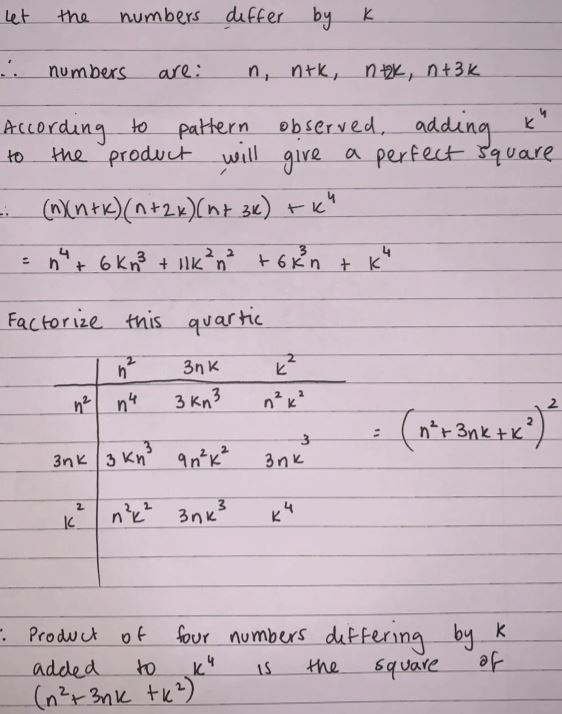Airi factorised the final expression so that it looks like Nikita's rule:

$\left(x^2+3kx+k^2\right)^2 = \left(x\left(x+3k\right)+\sqrt{k^4}\right)^2$

How could you factorise the final expression so that it looks like Isabel's rule or Sanika's rule?